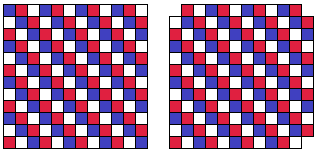# Tiling a 12x12 Square with Straight Trominoes

Consider a $12\times 12$ chessboard (consisting of $144$ $1\times 1$ squares.) If one removes 3 corners, can the remainder be covered by $47$ $1\times 3$ tiles (straight trominoes.)Solution### Solution

Consider a $12\times 12$ chessboard (consisting of $144$ $1\times 1$ squares.) If one removes 3 corners, can the remainder be covered by $47$ $1\times 3$ tiles (straight trominoes.)The answer to the question is negative. To see that, position the board as shown below, with the only remaining corner in the lower left. Color the board in three colors:Now, the rows 2-11 contain each exactly 40 squares of each of the colors. What is important is the number of squares of the three colors in the top and bottom rows. There are 8 red, 6 blue, and 7 white squares. However, every tromino - however placed on the board - covers exactly one square of each color. Thus it is impossible to cover the mutilated board with straight trominoes.

### References

1. M. I. Krusemeyer, G. T. Gilbert, L. C. Larson, A Mathematical Orchard, MAA, 2012, #33• Covering A Chessboard With Domino
• Dominoes on a Chessboard
• Tiling a Chessboard with Dominoes
• Vertical and Horizontal Dominoes on a Chessboard
• Straight Tromino on a Chessboard
• Golomb's inductive proof of a tromino theorem
• Tromino Puzzle: Interactive Illustration of Golomb's Theorem
• Tromino as a Rep-tile
• Tiling Rectangles with L-Trominoes
• Squares and Straight Tetrominoes
• Covering a Chessboard with a Hole with L-Trominoes
• Tromino Puzzle: Deficient Squares
• Tiling a Square with Tetrominoes Fault-Free
• Tiling a Square with T-, L-, and a Square Tetrominoes
• Tiling a Rectangle with L-tetrominoes
• Bicubal Domino
•